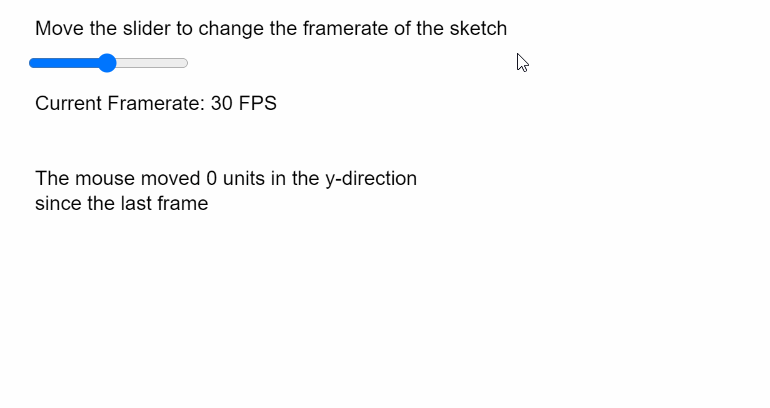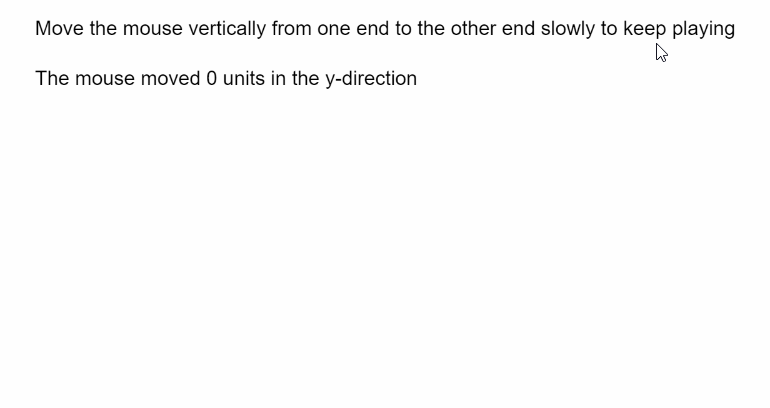Skip to content
Related Articles
p5.js | Mouse movedY
• Last Updated : 31 May, 2020

The movedY variable in p5.js contains the vertical movement of the mouse since the last frame of the sketch. A positive value indicates that the mouse moved down and a negative value indicates that it moved up in the last frame.

Syntax:

```movedY
```

The program below illustrates the movedY variable in p5.js:

Example 1:

## javascript

 `function` `setup() {``  ``createCanvas(400, 300);``  ``textSize(16);`` ` `  ``fpsSlider = createSlider(1, 60, 30, 1);``  ``fpsSlider.position(20, 40);``}`` ` `function` `draw() {``  ``clear();``  ``text(``"Move the slider to change the framerate of the sketch"``, 20, 20);``   ` `  ``// Set the framerate according to the slider``  ``frameRate(fpsSlider.value());``  ``text(``"Current Framerate: "` `+ fpsSlider.value() + ``" FPS"``, 20, 80);`` ` `  ``// Use the movedY property``  ``text(``"The mouse moved "` `+ movedY + ``" units in the y-direction"``, 20, 140);``  ``text(``"since the last frame"``, 20, 160);``}`

Output:Example 2:

## javascript

 `let gameOver = ``false``;`` ` `function` `setup() {``  ``createCanvas(600, 300);``  ``textSize(16);``}`` ` `function` `draw() {``  ``clear();``  ``text(``    ``"Move the mouse vertically from one end to the "``+``    ``"other end slowly to keep playing"``,``    ``20,``    ``20``  ``);``  ``if` `(!gameOver) {``    ``// Use the movedY property to display the``    ``// amount of mouse movedF``    ``text(``"The mouse moved "` `+ movedY + ``" units in "``+``         ``"the y-direction"``, 20, 60);`` ` `    ``// Get the absolute amount of mouse moved``    ``// and finish the game is it goes too fast``    ``if` `(abs(movedY) > 3) gameOver = ``true``;``  ``} ``else` `text(``"You moved too fast! Refresh to try again"``, 20, 80);``}`

Output:My Personal Notes arrow_drop_up